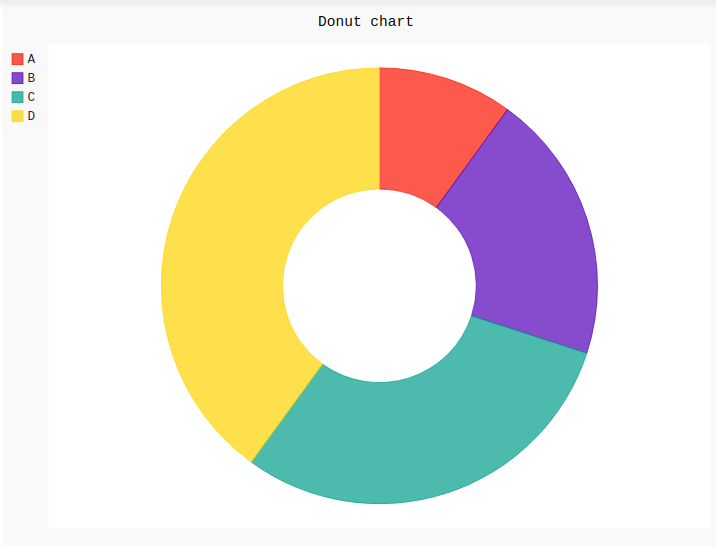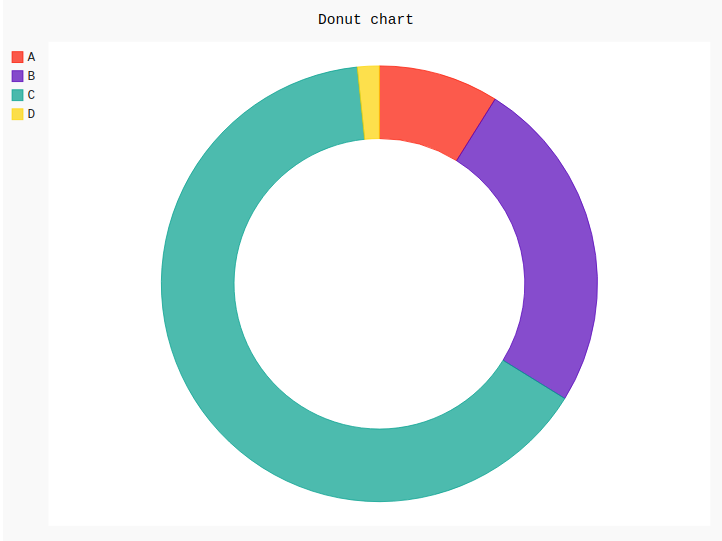# Donut chart in pygal

Pygal is a Python module that is mainly used to build SVG (Scalar Vector Graphics) graphs and charts. SVG is a vector-based graphics in the XML format that can be edited in any editor. Pygal can create graphs with minimal lines of code that can be easy to understand.

## Donut Chart

A donut pie chart essential a pie chart which has a hole in the center of the pie which makes the difference between the pie and the donut pie. This can be created using the Pie() method of the pygal module passing the parameter inner radius. The inner radius parameter should be set to a number between 0 and 1, commensurate to the ratio of radii between the hole and the chart.

Syntax:

`pie_chart = pygal.Pie(inner_radius=.4)`

Example 1:

 `# importing pygal ` `import` `pygal ` ` `  ` `  `# creating line chart object ` `pie_chart ``=` `pygal.Pie(inner_radius ``=` `.``4``) ` ` `  `# naming the title ` `pie_chart.title ``=` `'Donut chart'` ` `  `# random data ` `pie_chart.add(``'A'``, ``10``) ` `pie_chart.add(``'B'``, ``20``) ` `pie_chart.add(``'C'``, ``30``) ` `pie_chart.add(``'D'``, ``40``) ` ` `  `pie_chart `

Output:Example 2:

 `# importing pygal ` `import` `pygal ` ` `  ` `  `# creating line chart object ` `pie_chart ``=` `pygal.Pie(inner_radius ``=` `.``6``) ` ` `  `# naming the title ` `pie_chart.title ``=` `'Donut chart'` ` `  ` `  `# adding lines ` `pie_chart.add(``'A'``, ``115``) ` `pie_chart.add(``'B'``, ``322``) ` `pie_chart.add(``'C'``, ``834``) ` `pie_chart.add(``'D'``, ``21``) ` ` `  `pie_chart `

Output:My Personal Notes arrow_drop_upIf you like GeeksforGeeks and would like to contribute, you can also write an article using contribute.geeksforgeeks.org or mail your article to contribute@geeksforgeeks.org. See your article appearing on the GeeksforGeeks main page and help other Geeks.

Please Improve this article if you find anything incorrect by clicking on the "Improve Article" button below.

Article Tags :

Be the First to upvote.

Please write to us at contribute@geeksforgeeks.org to report any issue with the above content.